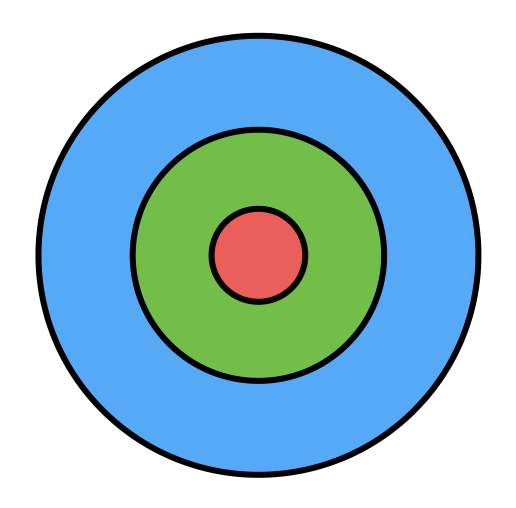Discrete Mathematics

# Geometric Probability: Level 2 ChallengesThe dartboard above is made up of three concentric circles with radii $$1, 3,$$ and $$5.$$ Assuming that a dart thrown will land randomly on the dartboard, what is the probability that it lands in the green region?

A point $$(a,b)$$ is randomly selected within the triangular region enclosed by the graphs of $$3y + 2x = 6$$, $$x = 0$$ and $$y = 0$$. What is the probability that $$b > a$$ ?

Give your answer as a decimal!

Two people stand around a circular duck pond which has radius $$R$$ meters. They are placed randomly (uniformly along the circumference of the duck pond) and independently.

What is the probability that they are more than $$R$$ meters apart from each other?

Dave and Kathy both arrive at Pizza Palace at two random times between 10:00 p.m. and midnight. They agree to wait exactly 15 minutes for each other to arrive before leaving. What is the probability that Dave and Kathy see each other?

If the probability is $$\frac ab$$ for coprime positive integers, give the answer as $$a+b$$.

A real number $$x$$ is chosen randomly and uniformly from the interval $$[2,10].$$ What is the probability that the greatest integer less than or equal to $$\frac{x}{2}$$ is even?

×

Problem Loading...

Note Loading...

Set Loading...